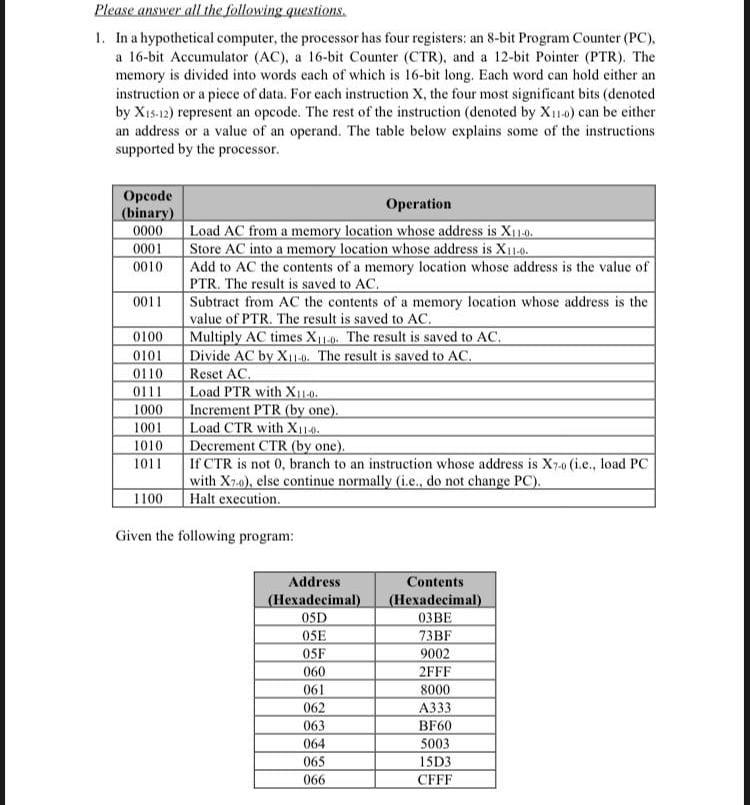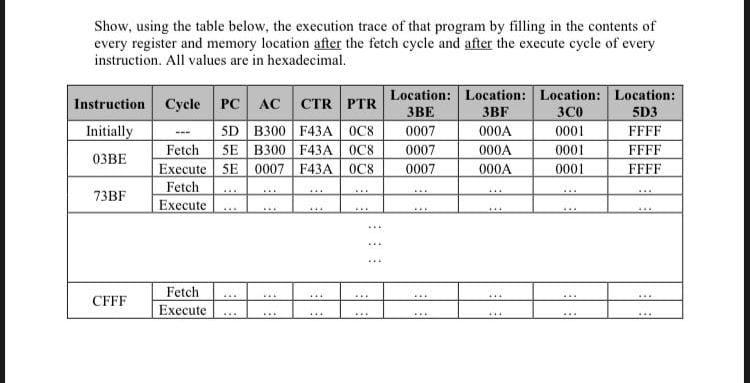Can someone here solve this assignment properly???????

Please answer all the following questions. 1. In a hypothetical computer, the processor has four registers: an 8-bit Program Counter (PC). a 16-bit Accumulator (AC), a 16-bit Counter (CTR), and a 12-bit Pointer (PTR). The memory is divided into words each of which is 16-bit long. Each word can hold either an instruction or a piece of data. For each instruction $$\mathrm{X}$$, the four most significant bits (denoted by $$\mathrm{X}_{15-12}$$ ) represent an opcode. The rest of the instruction (denoted by $$\mathrm{X}_{11-0}$$ ) can be either an address or a value of an operand. The table below explains some of the instructions supported by the processor. Given the following program: Show, using the table below, the execution trace of that program by filling in the contents of every register and memory location after the fetch cycle and after the execute cycle of every instruction. All values are in hexadecimal.

We have an Answer from Expert